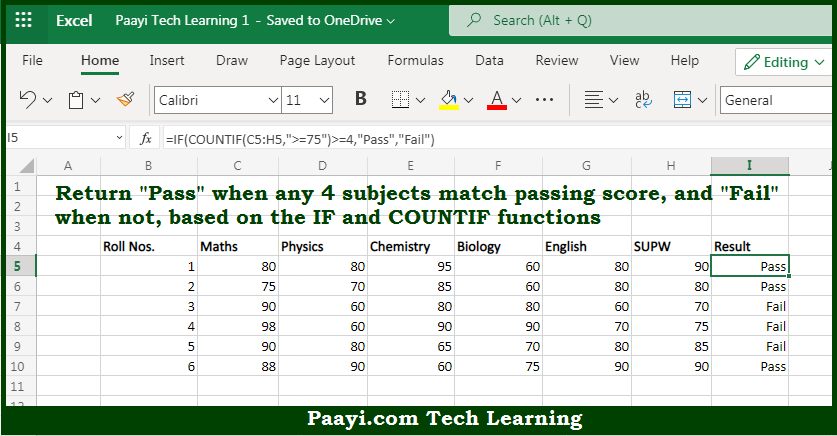# Learn How to Must Pass 4 Out of 6 in Microsoft Excel

Written by | 0 Comments | 594 Views

In this article, you will learn how to Must Pass 4 Out of 6 in Microsoft Excel using a single/combination(s) of functions. You will also know how to Must Pass 4 Out of 6 and see the generic formula.

Must Pass 4 Out of 6 in Microsoft Excel

The main purpose of this formula is to return "Pass" when any 4 subjects have a passing score, and "Fail" when not. Here we will learn how to get the must-pass 4 out of 6 results in the workbook in Microsoft Excel. That implies, with the help of a formula based on the IF and COUNTIF function you can able return "Pass" when any 4 subjects have a passing score, and "Fail" when not. So, with the help of this formula, you can able to get the must-pass 4 out of 6 results in the workbook in Microsoft Excel.

General Formula to Must Pass 4 Out of 6

=IF(COUNTIF(range,">=70")>=4,"Pass","Fail")

The Explanation for the Must Pass 4 Out of 6So we know that with the help of the given formula above you can able to return "Pass" when any 4 subjects have a passing score, and "Fail" when not. Here we will learn how to get the must-pass 4 out of 6 results in the workbook in Microsoft Excel. As we know that the number returned by the COUNTIF function is then checked against 4 with the greater than or equal to an operator (>=). After that, the expression returns TRUE or FALSE for the logical test inside the IF function. In case 4 or more subjects have a passing score of at least 70, IF returns "Pass". And in case not, the IF function returns "Fail". So, with the help of this formula, you can able to get the must-pass 4 out of 6 results in the workbook in Microsoft Excel.